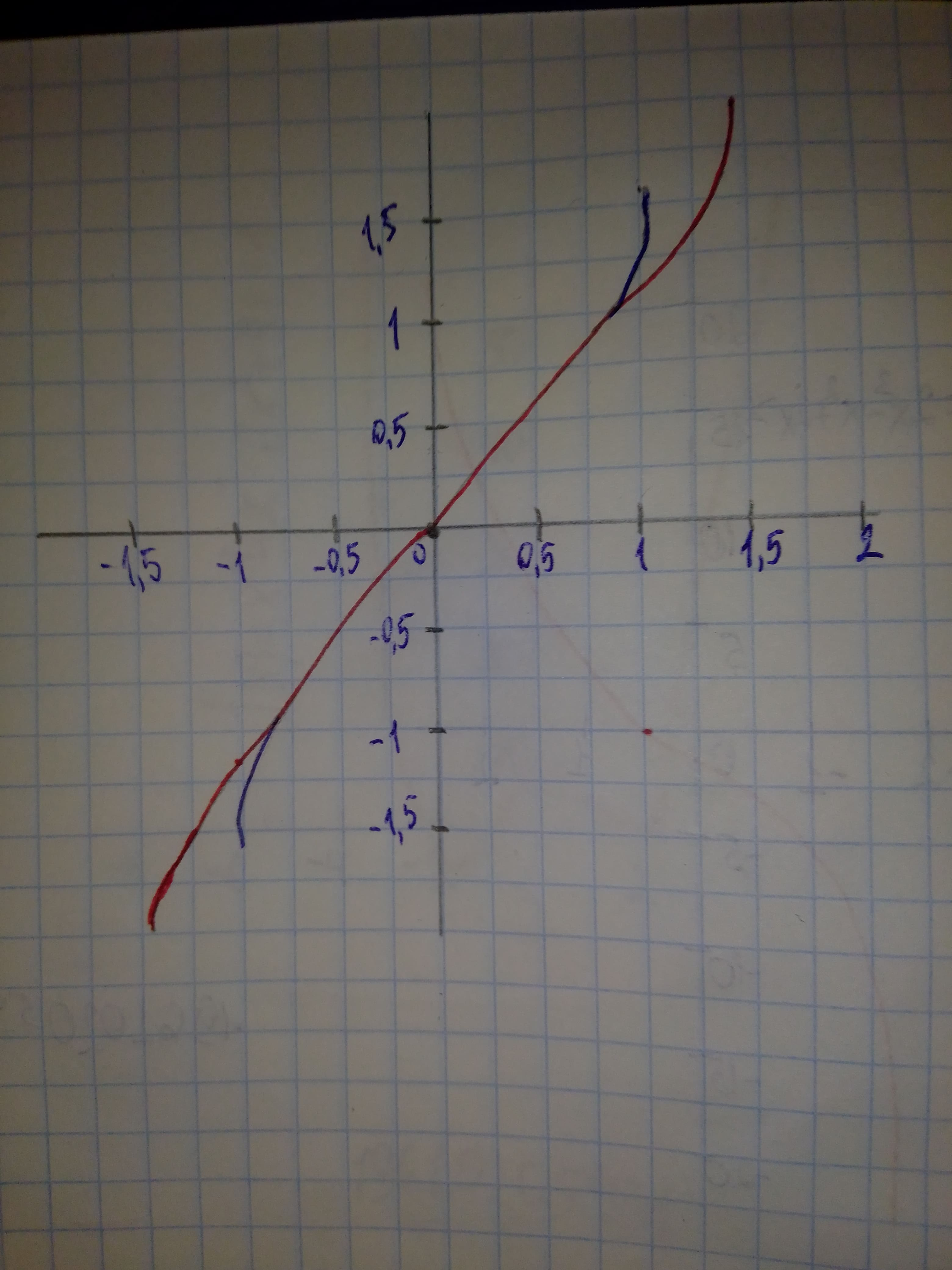# (a) find the Maclaurin polynomial P_{3}(x) for f(x), (b) complete the following x: -0.75, -0.50, -0.25, 0, 0.25, 0.50, 0.75 for f(x) and P_{3}(x), and (c) sketch the graphs of f(x) and P_{3}(x) on the same set of coordinate axes. f(x) = arcsin xvazelinahS 2020-12-15 Answered
(a) find the Maclaurin polynomial ${P}_{3}\left(x\right)$ for f(x), (b) complete the following $x:-0.75,-0.50,-0.25,0,0.25,0.50,0.75f\phantom{\rule{1em}{0ex}}\text{or}\phantom{\rule{1em}{0ex}}f\left(x\right)$ and ${P}_{3}\left(x\right)$, and (c) sketch the graphs of f(x) and ${P}_{3}\left(x\right)$ on the same set of coordinate axes. $f\left(x\right)=\mathrm{arcsin}x$
You can still ask an expert for help

• Questions are typically answered in as fast as 30 minutes

Solve your problem for the price of one coffee

• Math expert for every subject
• Pay only if we can solve itmhalmantus

a) $f\left(x\right)=\mathrm{arcsin}x$
${P}_{3}\left(x\right)=\sum _{k=0}^{3}\frac{{f}^{\left(k\right)}\left(0\right)}{k!}{x}^{k}$
${P}_{3}\left(x\right)=x+\frac{{x}^{3}}{6}$

$\begin{array}{|cccccccc|}\hline x& -0.75& -0.50& -0.25& -0.0& 0.25& 0.50& 0.75\\ f\left(x\right)& -0.848& -0.524& -0.253& 0& 0.253& 0.524& 0.848\\ {P}_{3}\left(x\right)& -0.820& -0.521& -0.253& 0& 0.253& 0.521& 0.820\\ \hline\end{array}$

On the graph below: Purple curve = $f\left(x\right)$ Orange curve = ${P}_{3}\left(x\right)$ Step 3